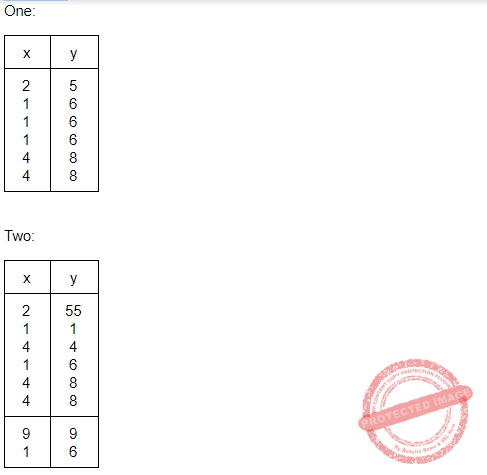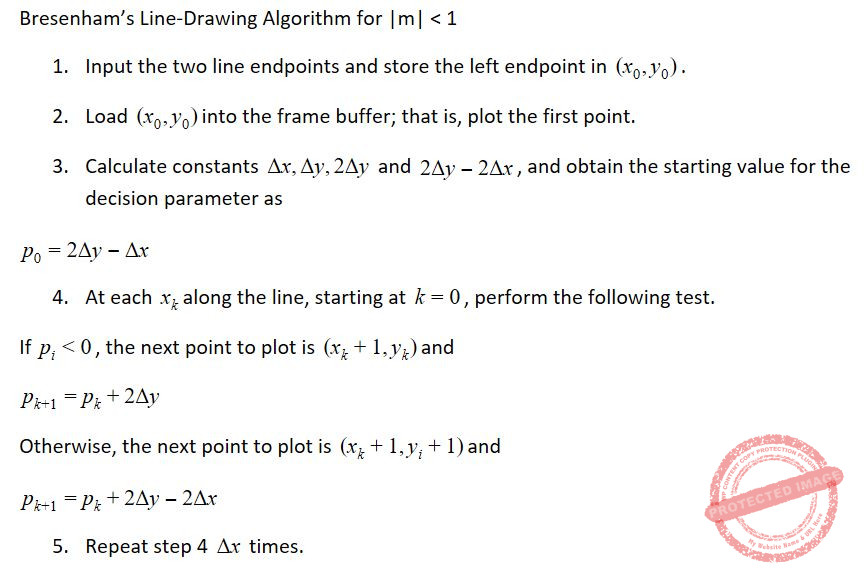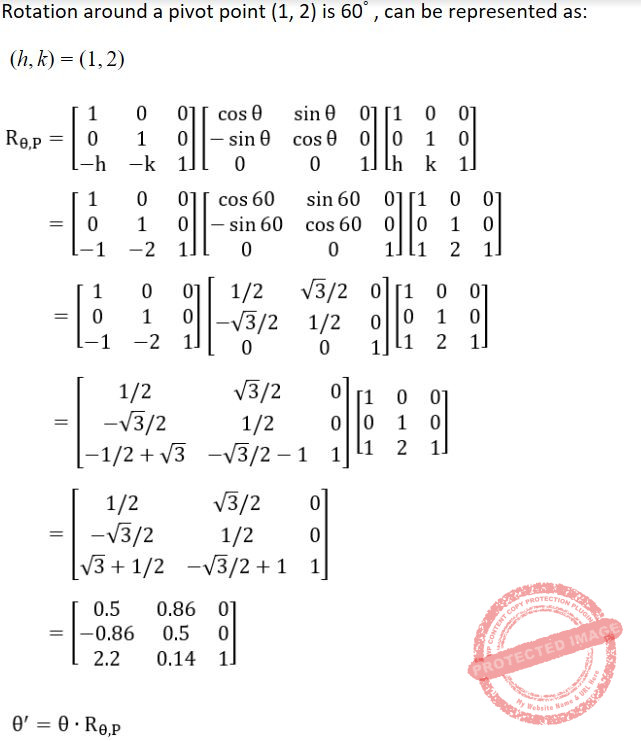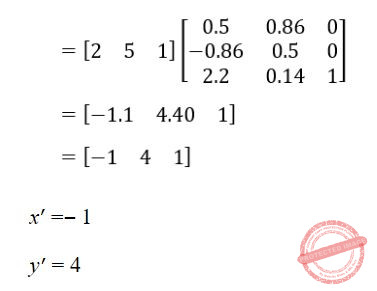## UGC NET CS 2016 Aug- paper-3

 Question 1
A ripple counter is a (n):
 A Synchronous Counter B Asynchronous counter C Parallel counter D None of the above
Digital-Logic-Design       Sequential-Circuits
 Question 2
8085 microprocessor has ____ bit ALU.
 A 32 B 16 C 8 D 4
Computer-Organization       Microprocessor
Question 2 Explanation:
8085 microprocessor has 8 bit ALU. It performs arithmetic and logical operations like Addition, Subtraction, AND, OR, etc. on 8-bit data.
 Question 3
The register that stores the bits required to mask the interrupts is ______.
 A Status register B Interrupt service register C Interrupt mask register D Interrupt request register
Computer-Organization       Interruption
Question 3 Explanation:
Status register(SR):
→ A status register is a collection of status flag bits for a processor.
→ The status register is a hardware register that contains information about the state of the processor. Individual bits are implicitly or explicitly read and/or written by the machine code instructions executing on the processor.
→ The status register lets an instruction take action contingent on the outcome of a previous instruction.
Interrupt service register(ISR):
→ Interrupt service routine(ISR) is a special block of code associated with a specific interrupt condition.
→ Interrupt handlers are initiated by hardware interrupts, software interrupt instructions, or software exceptions, and are used for implementing device drivers or transitions between protected modes of operation, such as system calls.
→ This register enables or masks interrupts from being triggered on the external pins of the Cache Controller.
→ The IMR is used to disable (Mask) or enable (Unmask) individual interrupt request inputs. This is also an 8-bit register.
Interrupt request register(IRR):
→ It stores all the interrupt inputs that are requesting service.
→ It is an 8-bit register, one bit for each interrupt request.
→ It keeps track of which interrupt inputs are asking for service. If an interrupt input is unmasked, and has an interrupt signal on it, then the corresponding bit in the IRR will be set.
→ The content of this register can be read to know the status of pending interrupts.
 Question 4
Which of the following in 8085 microprocessor performs
HL = HL + HL?
Computer-Organization       Microprocessor
 Question 5
In ______ addressing mode, the operands are stored in the memory. The address of the corresponding memory location is given in a register which is specified in the instruction.
 A Register direct B Register indirect C Base indexed D Displacement
Computer-Organization       Interruption
Question 5 Explanation:
Register indirect: It is specified implicitly in the definition of instruction. Register indirect addressing mode, the operands are stored in the memory. The address of the corresponding memory location is given in a register which is specified in the instruction.
Index mode: The address of the operand is obtained by adding to the contents of the general register (called index register) a constant value. The number of the index register and the constant value are included in the instruction code.
 Question 6
The output of the following combinational circuit.A X.Y B X+Y C X⊕Y D (X⊕Y)’
Digital-Logic-Design       Combinational-Circuit
Question 6 Explanation:Question 7
Which of the following statements is/are True regarding some advantages that an object-oriented DBMS (OODBMS) offers over a relational database ?
I. An OODBMS avoids the“impedance mismatch” problem.
II. An OODBMS avoids the “phantom” problem.
III. An OODBMS provides higher performance concurrency control than most relational databases.
IV. An OODBMS provides faster access to individual data objects once they have been read from disk.
 A II and III only B I and IV only C I, II, and III only D I, III and IV only
Database-Management-System       OODBMS
Question 7 Explanation:
TRUE: An OODBMS avoids the“impedance mismatch” problem.
FALSE: An OODBMS avoids the “phantom” problem.
FALSE: An OODBMS provides higher performance concurrency control than most relational databases.
TRUE: An OODBMS provides faster access to individual data objects once they have been read from disk.
 Question 8
The Global conceptual Schema in a distributed database contains information about global relations. The condition that all the data of the global relation must be mapped into the fragments, that is, it must not happen that a data item which belongs to a global relation does not belong to any fragment, is called:
 A Disjointness condition B Completeness condition C Reconstruction condition D Aggregation condition
Database-Management-System       Relational Schema
Question 8 Explanation:
Functional Completeness Condition: It is the condition used to check the equivalence(The condition that all the data of the global relation must be mapped into the fragments) between global relation and its fragments after decomposition. When a relation is decomposed into fragments, both global relation and its fragments should be able to determine each other.
For decomposing a global relation, lossless decomposition is the necessary condition but functionally completeness problem is not a necessary condition.
A relation in BCNF may or may not be functionally complete
 Question 9
Suppose database table T1(P, R) currently has tuples {(10, 5), (15, 8), (25, 6)} and table T2 (A, C) currently has {(10, 6), (25, 3), (10, 5)}. Consider the following three relational algebra queries RA1, RA2 and RA3:The number of tuples in the resulting table of RA1, RA2 and RA3 are given by:
 A 2, 4, 2 respectively B 2, 3, 2 respectively C 3, 3, 1 respectively D 3, 4, 1 respectively
Database-Management-System       Relational-Algebra
Question 9 Explanation:Question 10
Consider the table R with attributes A, B and C. The functional dependencies that hold on R are : A → B, C → AB. Which of the following statements is/are True ?
I. The decomposition of R into R1(C, A) and R2(A, B) is lossless.
II. The decomposition of R into R1(A, B) and R2(B, C) is lossy.
 A Only I B Only II C Both I and II D Neither I nor II
Database-Management-System       Functional-Dependency
Question 10 Explanation:Here ‘B’ is common attribute but ‘B’ is not the primary key in any of the decomposed relations (R1 or R2). Hence this decomposition is not a lossless decomposition i.e., it is a lossy decomposition.
Hence, option (C) is correct.
 Question 11
Consider the following ORACLE relations : One (x, y) = {<2, 5>, <1, 6>, <1, 6>, <1, 6>, <4, 8>, <4, 8>}
Two (x, y) = {<2, 55>, <1, 1>, <4, 4>, <1, 6>, <4, 8>, <4, 8>, <9, 9>, <1, 6>}
Consider the following two SQL queries SQ1 and SQ2 :
SQ1 : SELECT * FROM One)
EXCEPT
(SELECT * FROM Two);
SQ2 : SELECT * FROM One)
EXCEPT ALL
(SELECT * FROM Two);
For each of the SQL queries, what is the cardinality (number of rows) of the result obtained when applied to the instances above ?
 A 2 and 1 respectively B 1 and 2 respectively C 2 and 2 respectively D 1 and 1 respectively
Database-Management-System       SQL
Question 11 Explanation:
EXCEPT operation is like subtraction operation.
EXCEPT : EXCEPT operator do not include duplicates i.e if there are duplicate copies of a tuple in a relation the EXCEPT will consider only one copy of that duplicated tuple.
EXCEPT ALL : EXCEPT operator includes duplicates.Question 12
Which one of the following pairs is correctly matched in the context of database design?A I-D, II-A, III-B, IV-C B I-D, II-C, III-B, IV-A C I-C, II-D, III-A, IV-B D I-C, II-A, III-D, IV-B
Database-Management-System       Databases
Question 12 Explanation:
Specialization: Specialization is a top-down approach, means higher to lower. Result of taking a subset of a higher level entity set to from a lower level entity set.
Generalization: Generalization is a bottom-up approach, means lower to higher. Result of taking the union of two or more disjoint(lower-level) entity sets to produce higher-level entity set.
Aggregation: It is treated as higher level entity sets and can participate in relationships. Mapping cardinalities: Number of entities to which another entity can be associated via a relationship set. An abstraction in which relationship sets(along with their associated entity sets) are treated as higher level entity sets, and can participate in relationships.
Mapping cardinalities: Express the number of entities to which another entity can be associated via a relationship set.
 Question 13
Consider a raster grid having XY-axes in positive X-direction and positive upward Y-direction with Xmax = 10, Xmin = –5, Ymax = 11, and Ymin = 6. What is the address of memory pixel with location (5, 4) in raster grid assuming base address 1 (one) ?
 A 150 B 151 C 160 D 161
Computer-Graphics       Raster-Systems
 Question 14
Consider a N-bit plane frame buffer with W-bit wide lookup table with W > N. How many intensity levels are available at a time ?
 A 2N B 2 W C 2 N+W D 2 N-1
Computer-Graphics       Frame-Buffer
 Question 15
Consider the Bresenham's line generation algorithm for a line with gradient greater than one, current point (xi, yi) and decision parameter, di. The next point to be plotted (xi+1, yi+1) and updated decision parameter, di+1, for di < 0 are given as _______.
 A xi+1 = xi +1 yi+1 = yi di+1 = di+ 2 dy B xi+1 = xi yi+1 = yi +1 di+1 = di+ 2 dx C xi+1 = xi yi+1 = yi +1 di+1 = di+ 2(dx -dy) D xi+1 = xi +1 yi+1 = yi +1 di+1 = di+ 2(dy -dx)
Computer-Graphics       Bresenham’s-Algorithm
Question 15 Explanation:Question 16
A point P(2, 5) is rotated about a pivot point (1, 2) by 60°. What is the new transformed point P' ?
 A (1, 4) B (–1, 4) C (1, – 4) D (– 4, 1)
Computer-Graphics       Transformations
Question 16 Explanation:Question 17
In perspective projection (from 3D to 2D), objects behind the centre of projection are projected upside down and backward onto the view-plane. This is known as _____.
 A Topological distortion B Vanishing point C View confusion D Perspective foreshortening
Computer-Graphics       3D
 Question 18
The Liang-Barsky line clipping algorithm uses the parametric equation of a line from (x1, y1) to (x2, y2) along with its infinite extension which is given as :
x = x1 + ∆x.u
y = y1 + ∆y.u
Where ∆x = x2– x1, ∆y = y2– y1, and u is the parameter with 0 ≤ u ≤ 1. A line AB with endpoints A(–1, 7) and B(11, 1) is to be clipped against a rectangular window with xmin = 1, xmax = 9, ymin = 2, and ymax = 8.
The lower and upper bound values of the parameter u for the clipped line using Liang-Barsky algorithm is given as :
 A (0, 2/3) B (1/6 , 5/6) C (0, 1/3) D (0, 1)
Computer-Graphics       Liang-Barsky-line-clipping-algorithm
There are 18 questions to complete.

Register Now